# Brayton Cycle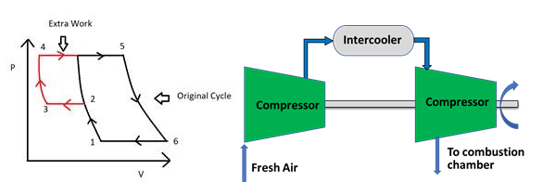# Brayton Cycle

The Brayton cycle is a thermodynamic cycle named after George Brayton that describes the workings of a constant-pressure heat engine. The Brayton cycle is the basis of the conventional gas turbine. The idea behind the Brayton Cycle is to extract energy from flowing air and fuel to generate usable work which can be used to power many vehicles by giving them thrust. Brayton Cycle is also called Gas Power Cycle.

The Brayton Cycle is a thermodynamic cycle that describes how gas turbines operate. This is a common cycle used in powerplants that operate using gas not steam. Gas power cycles are basically of two types: - Open and Closed Cycles. In the open cycle always fresh air is taken from the atmosphere and the exhaust is also discharged into the atmosphere as described in Fig.1, whereas in the closed cycle the same fluid is used again and again.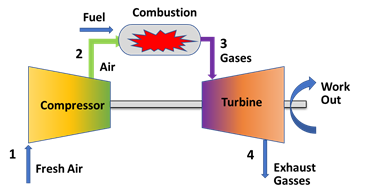Figure 1: Schematic representation of an open Brayton cycle

The cycle consists of four processes and is represented as:

1-2   Reversible Adiabatic Compression - the air is compressed from low pressure to high pressure in the compressor

2-3   Constant Pressure Heat Addition - heat is added to increase the enthalpy of gas either in the combustion chamber on the heat exchanger

3-4   Reversible Adiabatic Expansion - the air which has high enthalpy goes from high pressure to low pressure in the turbine as the expansion process takes place

4-1 Constant Pressure Heat Rejection - the heat is released to the atmosphere or the intercooler in the case of the open cycle and closed cycle respectively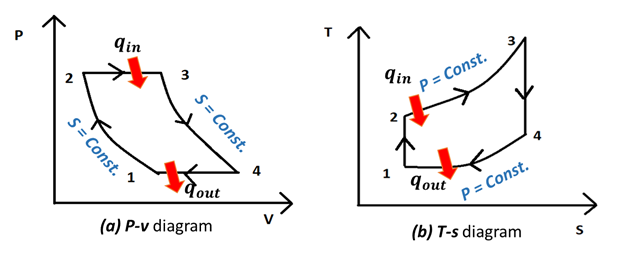Figure 2:  Brayton cycle process of P-v and T-s diagram

The 4-step process is represented by the above fig.2. Here mentioned the difference between open and closed cycles in a comparative way.

In the Open cycle, generally, the thermal efficiency comes out to be low, whereas in the closed cycle the efficiency is relatively high. There is a chance of erosion on the blades in the open cycle as the outside air enters the system regularly. But this problem is not posed in the closed cycle as the same fluid is operated and there is no undesired entry of moisture. This can pose a bigger problem as the turbine’s blades are very expensive and hence, they should be operated carefully. The open cycle is in most cases light-weighted than a closed system because it contains more sophisticated items which make it heavy. The application of the open cycle includes aircraft, stationary power plants, and automobiles. The application of the closed includes ships and submarines.

The assumptions of the air standard cycles are listed as follows:

1. The working substance is air, and its composition does not change
2. Specific heat values are considered constant irrespective of the temperature, hence it is assumed to be not affected by the temperature.
3. The change in the kinetic energy and the potential energy of the gas is considered to be negligible
4. During compression and the expansion process there is no heat loss to the surroundings
5. The loss of pressure is also not considered here

After analysis of the different processes, we will get a relation that the thermal efficiency of the Brayton cycle depends upon the pressure ratios and the nature of the gas. Figure 3.  depicts the relation of efficiency and pressure ratio for different gases and shows which one to be selected according to the requirement or need.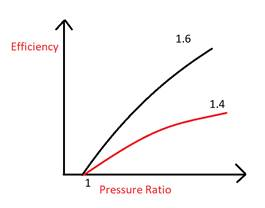Figure 3: show the efficiency and pressure ratio for different gases

There are several methods, how can be the efficiency of the Brayton cycle improved. These also aim to increase the work output and reduce the effort or work we are putting in the cycle. The most commonly used processes are as follows:

Regeneration: In this process, the exhaust gases which have a lot of heat inside them, can be utilized to increase the temperature of air coming out of the compressor. This will help us as we will have to provide less amount of heat to the gas in the combustion chamber. The effect of the regenerator is that the efficiency of the cycle increases as shown in the fig4(a). by putting in less amount of heat. Both the heat supplied and the heat rejected decrease. In this process, the mean temperature of the cycle also increases whereas the mean temperature of heat rejection decreases as in fig 4(b).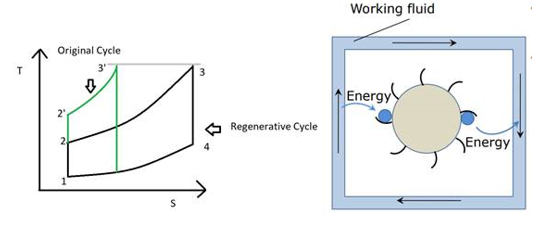Figure 4(a) & 4(b): Increased efficiency with regeneration and the application of practical example

Intercooling:  In this process, the temperature of the gas is reduced again and again with the help of a heat exchanger. The aim is to reduce the compressor work. Based on knowledge of thermodynamics, the slope of the adiabatic work is more as compared to isothermal. This means that if we try to find the flow work adiabatic process would have more work than isothermal work. So, in this process, we try to make it as close as the isothermal process so the compressor work could be saved as shown in fig. 5(a). If we have an infinite number of intercoolers in between we will approach the isothermal process as in fig.5(b). The effects of the intercooler are that both heat supplied and heat rejected increases. Also, the mean temperature of heat addition and mean temperature of heat rejection decreases. For the same output, the plant becomes compact as the mass flow rate decreases. Combinations of these are often used to create the appropriate cycles.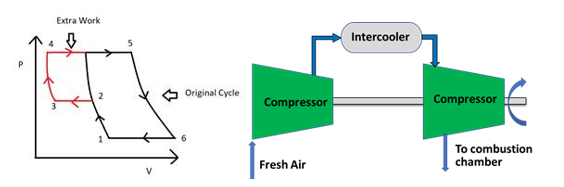Figure 5(a) & 5(b): Increased efficiency with intercooling and the application in gas turbine

Reheating: - Here network output is increased by increasing the work done by a turbine and it is obtained by using several stages of expansion with heating in between the stages. The effects of reheating include the increase of turbine output; Hence the net work increases. Both heats supplied and the heat rejected increases. The most important part of reheating is that because of it the mean temperature of the heat addition and heat rejection increases. But the cycle efficiency decreases as the mean temperature of heat rejection increases as shown in fig.6(a). Also, the scope of heat regeneration increases because turbine exit temperature increases as shown in fig. 6(b).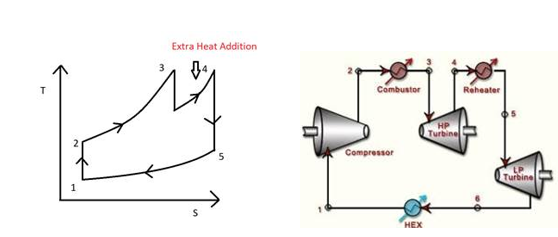Figure 6(a) & (b): Increased efficiency with intercooling and the application of practical example

Gas turbines are the best examples that operate based on Brayton open cycle. Air at ambient conditions is drawn into the compressor, where its temperature and pressure are raised. The high-pressure air proceeds into the combustion chamber, where the fuel is burned at constant pressure.  The high-temperature gases then enter the turbine where they expand to atmospheric pressure while producing power output. Some of the output power is used to drive the compressor. The exhaust gases leaving the turbine are thrown out (not re-circulated), causing the cycle to be classified as an open cycle as shown in fig.7 and the efficiency i.e., T-s diagram of an ideal gas turbine cycle with a regenerator, reheating and intercooling are as shown in fig. 8.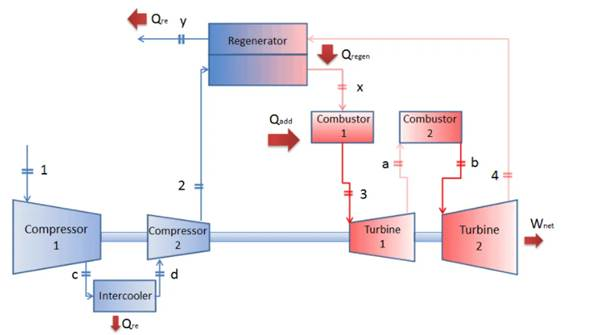Figure 7: Process of gas turbine -open cycle with regenerator and intercooler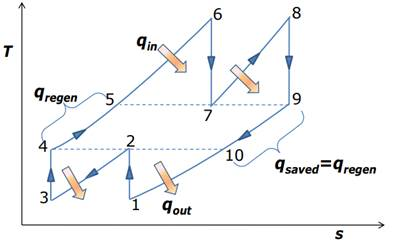Figure 8: T-s diagram of an ideal gas turbine cycle with a regenerator, reheating & intercooling

The open gas-turbine cycle can be modeled as a closed cycle, using the air-standard assumption. The compression and expansion processes remain the same, but the combustion process is replaced by a constant-pressure heat addition process from an external source. The exhaust process is replaced by a constant-pressure heat rejection process to the ambient air.

To conclude, Intercooling and reheating always decrease thermal efficiency unless are accompanied by regeneration. Therefore, in gas turbine power plants, intercooling and reheating are always used in conjunction with regeneration.

References:

Related Blog1 year ago / 0

Supports are arguably one of the most important aspects of a structure, as it specifies how the forc…1 year ago / 0

Petrol Vs Diesel Engine Engines are the powerhouse of vehicles and systems. It provides the energ…